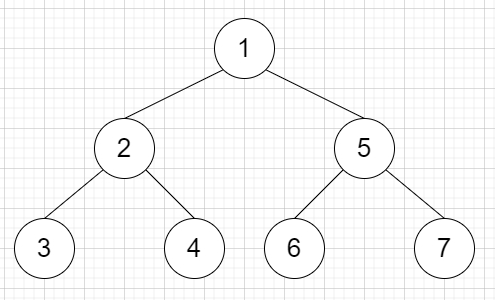# Recover a Tree From Preorder Traversal in C++

Suppose there is a binary tree. We will run a preorder depth first search on the root of a binary tree.

At each node in this traversal, the output will be D number of dashes (Here D is the depth of this node), after that we display the value of this node. As we know if the depth of a node is D, the depth of its immediate child is D+1 and the depth of the root node is 0.

Another thing we have to keep in mind that if a node has only one child, that child is guaranteed to be the left child. So, if the output S of this traversal is given, then recover the tree and return its root.

So, if the input is like "1-2--3--4-5--6--7", then the output will beTo solve this, we will follow these steps −

• Define one stack st

• i := 0, n := size of S

• lvl := 0, num := 0

• while i < n, do −

• for initialize lvl := 0, when S[i] is same as '-', update (increase lvl by 1), (increase i by 1), do −

• do nothing

• num := 0

• while (i < n and S[i] is not equal to '-'), do −

• num := num * 10 + (S[i] - '0')

• (increase i by 1)

• while size of st > lvl, do −

• delete element from st

• temp = create a new Tree Node with num value

• if not st is empty and not left of top element of st is null, then −

• left of top element of st := temp

• otherwise when not st is empty, then −

• right of top element of st := temp

• insert temp into st

• while size of st > 1, do −

• delete element from st

• return (if st is empty, then NULL, otherwise top element of st)

Let us see the following implementation to get better understanding −

## Example

Live Demo

#include <bits/stdc++.h>
using namespace std;
class TreeNode{
public:
int val;
TreeNode *left, *right;
TreeNode(int data){
val = data;
left = NULL;
right = NULL;
}
};
void inord(TreeNode *root){
if(root != NULL){
inord(root->left);
cout << root->val << " ";
inord(root->right);
}
}
class Solution {
public:
TreeNode* recoverFromPreorder(string S) {
stack<TreeNode*> st;
int i = 0;
int n = S.size();
int lvl = 0;
int num = 0;
while (i < n) {
for (lvl = 0; S[i] == '-'; lvl++, i++)
;
num = 0;
while (i < n && S[i] != '-') {
num = num * 10 + (S[i] - '0');
i++;
}
while (st.size() > lvl)
st.pop();
TreeNode* temp = new TreeNode(num);
if (!st.empty() && !st.top()->left) {
st.top()->left = temp;
}
else if (!st.empty()) {
st.top()->right = temp;
}
st.push(temp);
}
while (st.size() > 1)
st.pop();
return st.empty() ? NULL : st.top();
}
};
main(){
Solution ob;
TreeNode *root = ob.recoverFromPreorder("1-2--3--4-5--6--7");
inord(root);
}

## Input

"1-2--3--4-5--6--7"

## Output

3 2 4 1 6 5 7